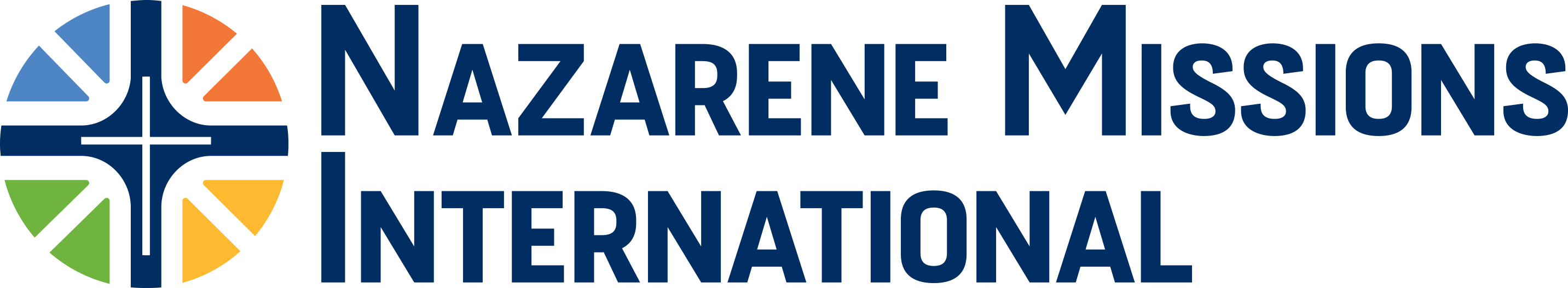### Question Title

#### * 3. NMI Membership (as reported on the APR)

 0-37 NMI members = 2 delegates 313-337 = 14 613-637 = 26 38-62 = 3 338-362 = 15 638-662 = 27 63-87 = 4 363-387 = 16 663-687 = 28 88-112 = 5 388-412 = 17 688-712 = 29 113-137 = 6 413-437 = 18 713-737 = 30 138-162 = 7 438-462 = 19 738-762 = 31 163-187 = 8 463-487 = 20 763-787 = 32 188-212 = 9 488-512 = 21 788-812 = 33 213-237 = 10 513-537 = 22 813-837 = 34 238-262 = 11 538-562 = 23 838-862 = 35 263-287 = 12 563-587 = 24 863-887 = 36 288-312 = 13 588-612 = 25 888-912 = 37

T